## TIFR PHD CS & SS 2017

 Question 1
A suitcase weighs one kilogram plus half of its weight. How much does the suitcase weigh?
 A 1.333... kilograms B 1.5 kilograms C 1.666... kilograms D 2 kilograms E cannot be determined from the given data
Aptitude       Numerical
 Question 2A (a, b)≤ ǁbǁ B (a, b)≤ ǁaǁ C (a, b) = ǁaǁǁbǁ D (a, b)≥ ǁbǁ E (a, b)≥ ǁaǁ
Engineering-Mathematics       Vectors
 Question 3
On planet TIFR, the acceleration of an object due to gravity is half that on planet earth. An object on planet earth dropped from a height h takes time t to reach the ground. On planet TIFR, how much time would an object dropped from height h take to reach the ground?
 A t/√2 B √2t C 2t D h/t E h/2t
Aptitude       Numerical
 Question 4
Which of the following functions asymptotically grows the fastest as n goes to inﬁnity?
 A (log log n)! B (log log n)log n C (log log n)log log log n D (log n)log log n E 2√(log log n)
Algorithms       Asymptotic-Complexity
 Question 5
How many distinct ways are there to split 50 identical coins among three people so that each person gets at least 5 coins?
 A 335 B 350 − 250 C (35 2) D (50 15)*335 E (37 2)
Engineering-Mathematics       Combinatorics
 Question 6
How many distinct words can be formed by permuting the letters of the word ABRACADABRA ?
 A 11! / (5! 2! 2!) B 11! / (5! 4!) C 11! 5! 2! 2! D 11! E 11! 5! 4!
Engineering-Mathematics       Combinatorics
 Question 7
Consider the sequence S0, S1, S2,... deﬁned as follows: S0 = 0, S1 = 1, and Sn = 2Sn−1 + Sn−2 for n ≥ 2. Which of the following statements is FALSE?
 A for every n ≥ 1, S2n is even B for every n ≥ 1, S2n+1 is odd C for every n ≥ 1, S3n is a multiple of 3 D for every n ≥ 1, S4n is a multiple of 6 E none of the above
Algorithms       Recurrences
 Question 8
In a tutorial on geometrical constructions, the teacher asks a student to construct a right-angled triangle ABC where the hypotenuse BC is 8 inches and the length of the perpendicular dropped from A onto the hypotenuse is h inches, and oﬀers various choices for the value of h. For which value of h can such a triangle NOT exist?
 A 3.90 inches B 2√2 inches C 2√3 inches D 4.1 inches E none of the above
Aptitude       Numerical
 Question 9A 3α B α2 C 6α(1 − α) D 3α2(1 − α) E 6α(1 − α)+ α2
Engineering-Mathematics       Probability-and-statistics
 Question 10
For a set A, deﬁne (A) to be the set of all subsets of A. For example, if A = { 1, 2 }, then P(A) = {Φ,{1, 2},{1},{2}}. Let f : A -->P(A) be a function and A is not empty. Which of the following must be TRUE?
 A f cannot be one-to-one (injective) B f cannot be onto (surjective) C f is both one-to-one and onto (bijective) D there is no such f possible E if such a function f exists, then A is inﬁnite
Engineering-Mathematics       Relations-and-Functions
 Question 11
Let fog denote function composition such that (fog)(x) = f (g(x)). Let f :A --> B such that for all g : B --> A and h : B-->A we have fog = foh ==> g=h. Which of the following must be TRUE?
 A f is onto (surjective) B f is one-to-one (injective) C f is both one-to-one and onto (bijective) D the range of f is ﬁnite E the domain of f is ﬁnite
Engineering-Mathematics       Relations-and-Functions
 Question 12
Consider the following program modifying an n × n square matrix A:
for i = 1 to n:
for j = 1 to n:
temp = A[i][j] + 10 A[i][j] = A[j][i]
A[j][i] = temp - 10 end for
end for
Which of the following statements about the contents of the matrix A at the end of this program must be TRUE ?
 A the new A is the transpose of the old A B all elements above the diagonal have their values increased by 10 and all values below have their values decreased by 10 C all elements above the diagonal have their values decreased by 10 and all values below have their values increased by 10 D the new matrix A is symmetric, that is, A[i][j] = A[j][i] for all 1 ≤ i, j ≤ n E A remains unchanged
Programming       Control-Statement
 Question 13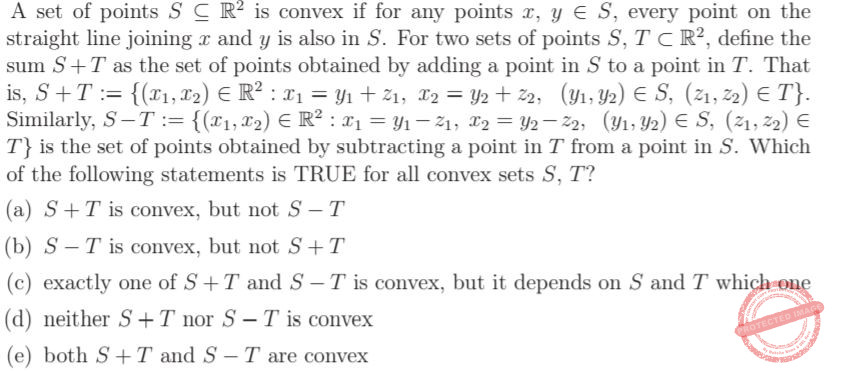A a B b C c D d E e
Engineering-Mathematics       Set-Theory
 Question 14
Consider the following game with two players, Aditi and Bharat. There are n tokens in a bag. The players know n, and take turns removing tokens from the bag. In each turn, a player can either remove one token or two tokens. The player that removes the last token from the bag loses. Assume that Aditi always goes ﬁrst. Further, we say that a player has a winning strategy if she or he can win the game, no matter what the other player does. Which of the following statements is TRUE?
 A For n = 3, Bharat has a winning strategy. For n = 4, Aditi has a winning strategy B For n = 7, Bharat has a winning strategy. For n = 8, Aditi has a winning strategy C For both n = 3 and n = 4, Aditi has a winning strategy D For both n = 7 and n = 8, Bharat has a winning strategy E Bharat never has a winning strategy
Aptitude       Numerical
 Question 15A a B b C c D d E e
Algorithms       Recurrences
 Question 16A 39 B 63 C 3*28 D 27 E 24
Engineering-Mathematics       Graph-Theory
 Question 17
Consider the following statements:
(i) Checking if a given undirected graph has a cycle is in P.
(ii) Checking if a given undirected graph has a cycle is in NP.
(iii) Checking if a given directed graph has a cycle is in P.
(iv) Checking if a given directed graph has a cycle is in NP.
Which of the above statements is/are TRUE? Choose from the following options.
 A Only (i) and (ii) B Only (ii) and (iv) C Only (ii), (iii), and (iv) D Only (i), (ii), and (iv) E All of them
Algorithms       P-NP
 Question 18
We have an implementation that supports the following operations on a stack (in the instructions below, s is the name of the stack).
isempty(s): returns True if s is empty, and False otherwise .
top(s): returns the top element of the stack, but does not pop the stack; returns
null if the stack is empty.
push(s,x): places x on top of the stack.
pop(s): pops the stack; does nothing if s is empty. Consider the following code:
pop_ray_pop(x): s = empty
for i = 1 to length(x): if (x[i] == ’(’):
push(s,x[i]) else:
while (top(s) == ’(’): pop(s)
end while push(s, ’)’)
end if end for
while not isempty(s): print top(s) pop(s)
end while
What is the output of this program when pop_ray_pop("(((()((())((((") is executed ?
 A (((( B )))(((( C ))) D (((())) E ()()
Data-Structures       Queues-and-Stacks
 Question 19
Let L be the language over the alphabet {1, 2, 3, (, )} generated by the following grammar (with start symbol S, and non-terminals {A, B, C}): S → AB C A → ( B → 1B | 2B | 3B B → 1 | 2 | 3 C → ) Then, which of the following is TRUE?
 A L is ﬁnite B L is not recursively enumerable C L is regular D L contains only strings of even length E L is context-free but not regular
Theory-of-Computation       Languages-and-Grammars
 Question 20
Consider the following pseudocode fragment, where y is an integer that has been initialized. int i = 1 int j = 1 while (i < 10): j = j * i i = i + 1 if (i==y): break end if end while Consider the following statements: (i) (i == 10) or (i == y) (ii) If y > 10, then i == 10 (iii) If j = 6, then y == 4 Which of the above statements is/are TRUE at the end of the while loop? Choose from the following options.
 A (i) only B (iii) only C (ii) and (iii) only D (i), (ii), and (iii) E None of the above
Programming       Control-Statement
 Question 21
Consider First Order Logic (FOL) with equality and suitable function and relation symbols. Which one of the following is FALSE?
 A Partial orders cannot be axiomatized in FOL B FOL has a complete proof system C Natural numbers cannot be axiomatized in FOL D Real numbers cannot be axiomatized in FOL E Rational numbers cannot be axiomatized in FOL
Engineering-Mathematics       Sets-And-Relation
 Question 22
An array of n distinct elements is said to be un-sorted if for every index i such that 2 <=i <=n-1, either A[i] > max{A[i-1], A[i + 1] , or A[i] < min A[i-1], A[i + 1] . What is the time-complexity of the fastest algorithm that takes as input a sorted array A with n distinct elements, and un-sorts A?
 A O(n log n) but not O(n) B O(n) but not O(√n) C O(√n) but not O(log n) D O(log n) but not O(1) E O(1)
Algorithms       Sorting
 Question 23
For any natural number n, an ordering of all binary strings of length n is a Gray code if it starts with 0n, and any successive strings in the ordering diﬀer in exactly one bit (the ﬁrst and last string must also diﬀer by one bit). Thus, for n = 3, the ordering (000, 100, 101, 111, 110, 010, 011, 001) is a Gray code. Which of the following must be TRUE for all Gray codes over strings of length n?
 A the number of possible Gray codes is even B the number of possible Gray codes is odd C in any Gray code, if two strings are separated by k other strings in the ordering, then they must diﬀer in exactly k +1 bits D in any Gray code, if two strings are separated by k other strings in the ordering, then they must diﬀer in exactly k bits E none of the above
Digital-Logic-Design       Number-Systems
 Question 24
Which of the following regular expressions correctly accepts the set of all 0/1-strings with an even (possibly zero) number of 1s?
 A (10∗10∗)∗ B (10∗10∗1)∗ C 0∗1(10∗1)∗10∗ D 0∗1(0∗10∗10∗)∗10∗ E (0∗10∗1)∗0∗
Theory-of-Computation       Regular-Expression
 Question 25
A vertex colouring of a graph G = (V, E) with k colours is a mapping c : V-->{1,..., k} such that c(u) ≠ c(v) for every (u, v) ϵ E. Consider the following statements: (i) If every vertex in G has degree at most d, then G admits a vertex colouring using d+1 colours. (ii) Every cycle admits a vertex colouring using 2 colours. (iii) Every tree admits a vertex colouring using 2 colours. Which of the above statements is/are TRUE? Choose from the following options.
 A only (i) B only (i) and (ii) C only (i) and (iii) D only (ii) and (iii) E (i), (ii), and (iii)
Engineering-Mathematics       Graph-Theory
 Question 26
Given that
B(x) means “x is a bat”,
F (x) means “x is a ﬂy”, and
E(x, y) means “x eats y”,
what is the best English translation of
∀x(F(x) → ∀y(E(y, x) → B(y)))?
 A all ﬂies eat bats B every ﬂy is eaten by some bat C bats eat only ﬂies D every bat eats ﬂies E only bats eat ﬂies
Engineering-Mathematics       Propositional-Logic
 Question 27
An undirected graph is complete if there is an edge between every pair of vertices. Given a complete undirected graph on n vertices, in how many ways can you choose a direction for the edges so that there are no directed cycles?
 A n B n(n-1)/2 C n! D 2n E 2m ,where m=n(n-1)/2
Engineering-Mathematics       Graph-Theory
 Question 28
For an undirected graph G = (V, E), the line graph G∗ = (V ∗, E∗) is obtained by replacing each edge in E by a vertex, and adding an edge between two vertices in V ∗ if the corresponding edges in G are incident on the same vertex. Which of the following is TRUE of line graphs?
 A the line graph for a complete graph is complete B the line graph for a connected graph is connected C the line graph for a bipartite graph is bipartite D the maximum degree of any vertex in the line graph is at most the maximum degree in the original graph E each vertex in the line graph has degree one or two
Engineering-Mathematics       Graph-Theory
 Question 29
Consider the following grammar G with terminals {[, ]}, start symbol S, and non- terminals {A, B, C}:
S → AC | SS | AB C → SB
A → [
B → ]
A language L is called preﬁx-closed if for every xεL, every preﬁx of x is also in L. Which of the following is FALSE?
 A L(G) is context free B L(G) is inﬁnite C L(G) can be recognized by a deterministic push down automaton D L(G) is preﬁx-closed E L(G) is recursive.
Theory-of-Computation       Languages-and-Grammars
 Question 30
A multivariate polynomial in n variables with integer coeﬃcients has a binary root if it is possible to assign each variable either 0 or 1, so that the polynomial evaluates to 0. For example, the multivariate polynomial -2x31-x1x1+2 has the binary root (x1 = 1, x1 = 0). Then determining whether a multivariate polynomial, given as the sum of monomials, has a binary root:
 A is trivial: every polynomial has a binary root B can be done in polynomial time C is NP-hard, but not in NP D is in NP, but not in P and not NP-hard E is both in NP and NP-hard
Engineering-Mathematics       Polynomials
 Question 31
Consider a system which in response to input x(t) outputs
y(t) = 2x(t − 2) + x(2t − 1) + 1.
Which of the following describes the system?
 A linear, time-invariant, causal B linear, time-invariant, non-causal C non-linear, time-invariant, causal D non-linear, time-invariant, non-causal E non-linear, time-variant
 Question 32
Suppose a 1μH inductor and a 1Ω resistor are connected in series to a 1V battery. What happens to the current in the circuit?
 A The current starts at 0A, and gradually rises to 1A B The current rises instantaneously to 1A and stays there after that C The current rises instantaneously to 1A and then falls to 0A D The current oscillates over time between 0A and 1A E The current oscillates over time between 1A and -1A
 Question 33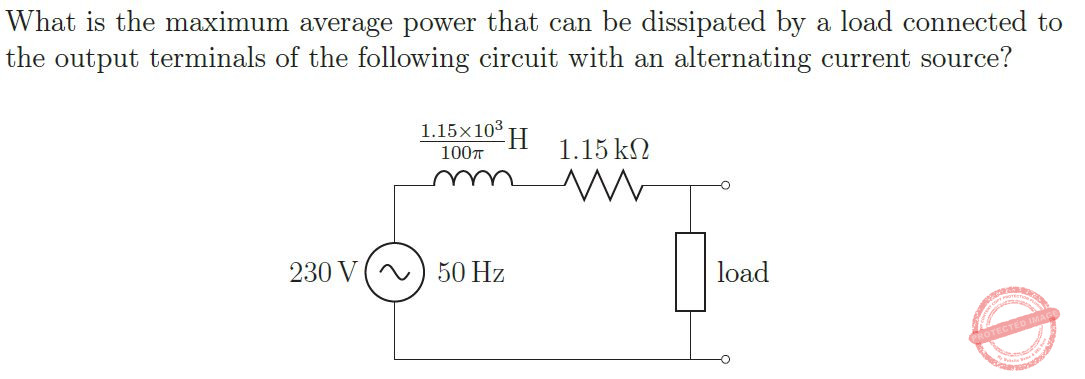A 23 W B 11.5W C 8.1317W D 2.875W E None of the above
 Question 34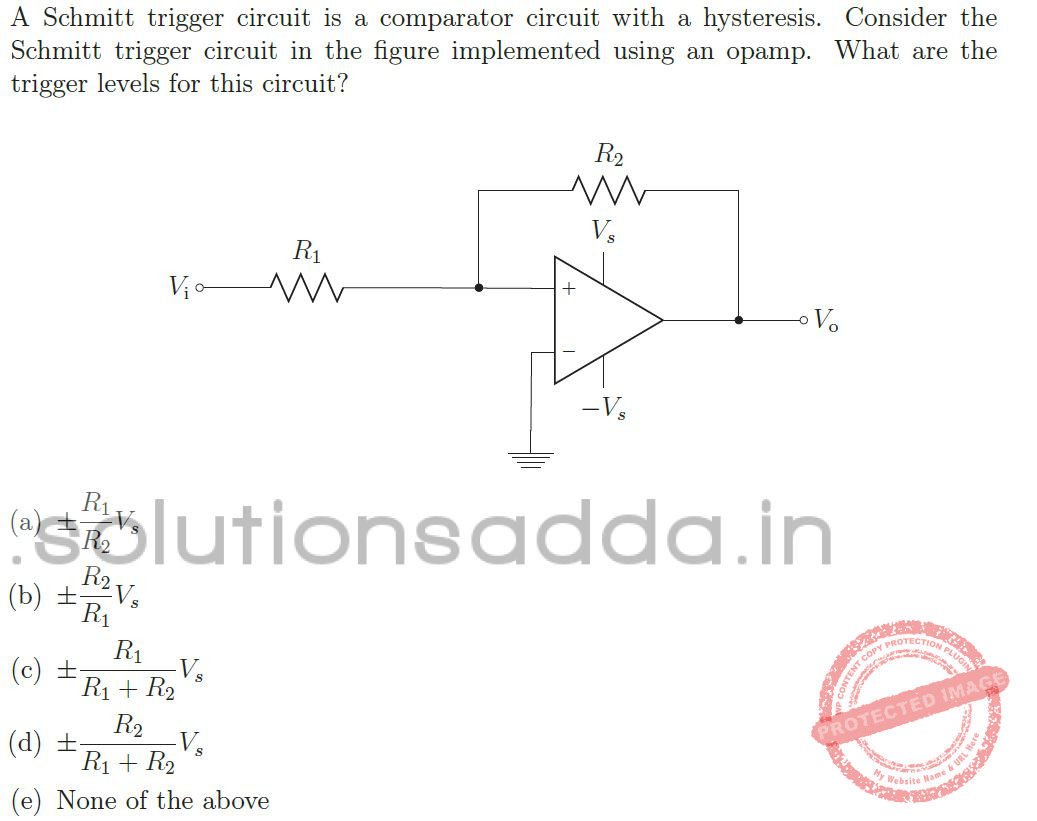A a B b C c D d E e
 Question 35A a B b C c D d E e
Engineering-Mathematics       Linear-Inequality
 Question 36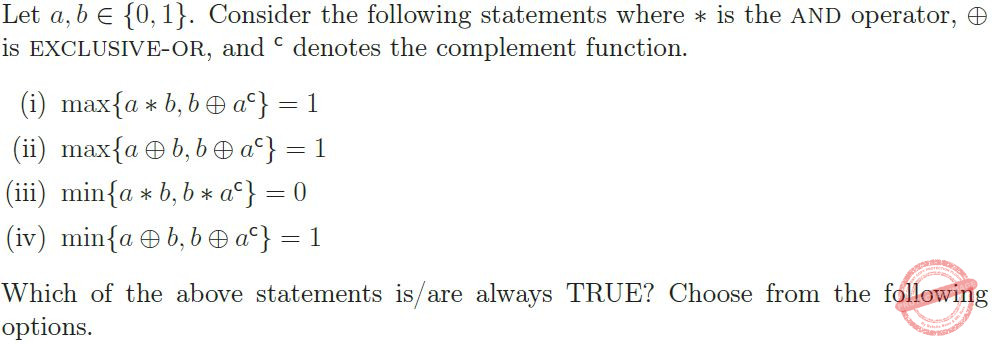A (i) and (ii) only B (ii) and (iii) only C (iii) and (iv) only D (iv) and (i) only E None of the above
Digital-Logic-Design       Logic-Gates-and-operators
 Question 37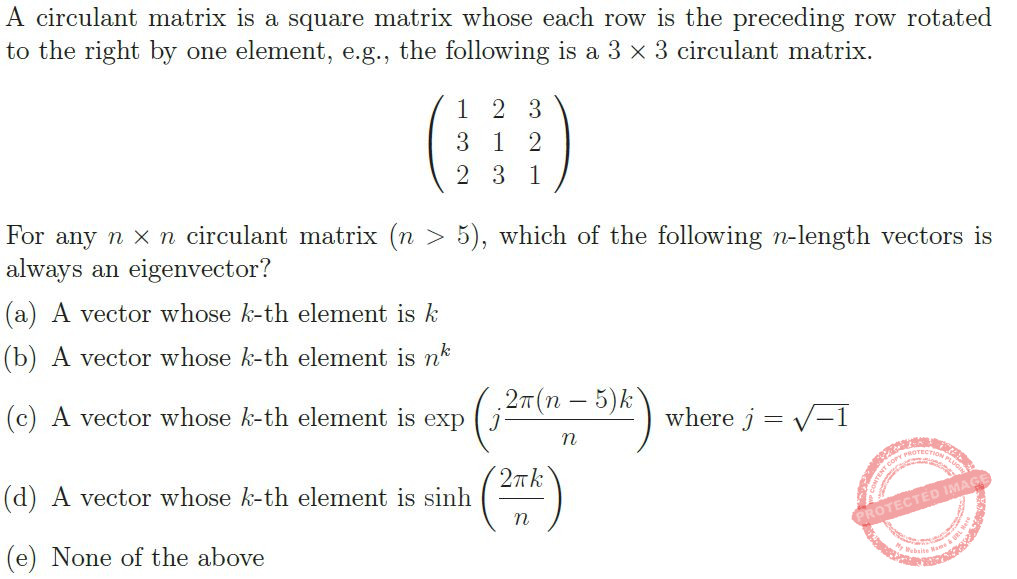A a B b C c D d E e
Engineering-Mathematics       Linear-Algebra
 Question 38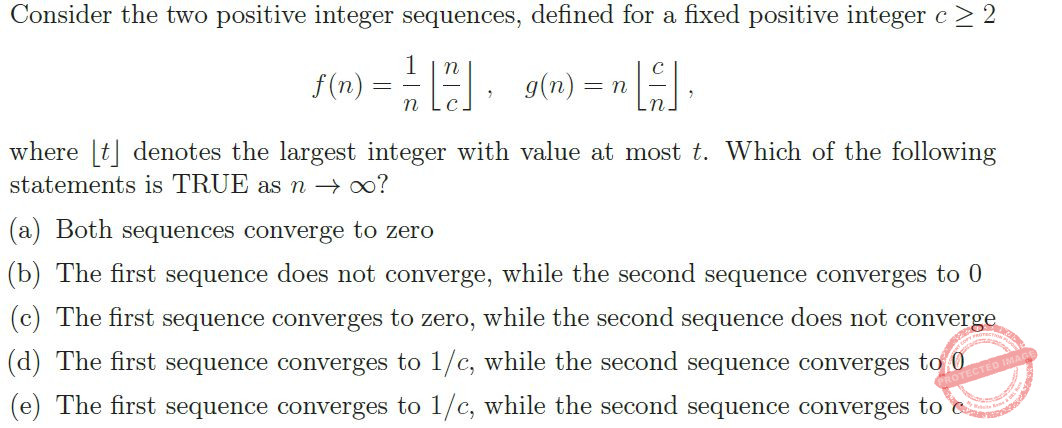A a B b C c D d E e
Engineering-Mathematics       Calculus
 Question 39A a B b C c D d E e
Engineering-Mathematics       Probability-and-statistics
 Question 40A a B b C c D d E e
Engineering-Mathematics       Probability-and-statistics
 Question 41
Consider a unit length interval [0, 1] and choose a point X on it with uniform distribution. Let L1 = X and L2 = 1-X be the length of the two sub-intervals created by this point on the unit interval. Let L = max L1, L2 . Consider the following statements where E denotes expectation.
(i) E[L] = 3/4
(ii) E[L] = 2/3
(iii) L is uniformly distributed over [1/2, 1]
(iv) L is uniformly distributed over [1/3, 1]
Which of the above statements is/are TRUE? Choose from the following options.
 A Only (i) B Only (ii) C Only (i) and (iii) D Only (ii) and (iv) E None of the above
Engineering-Mathematics       Probability-and-statistics
 Question 42A Only (i) B Only (ii) C Only (iii) D Only (i) and (ii) E Only (i) and (iii)
Engineering-Mathematics       Probability-and-statistics
 Question 43A Only (i) B Only (ii) C Only (iii) D (i), (ii), and (iii) E None of the above
Engineering-Mathematics       Linear-Algebra
 Question 44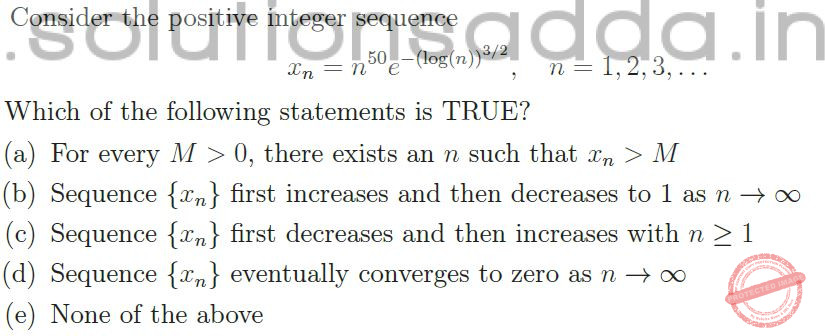A a B b C c D d E e
Engineering-Mathematics       Calculus
 Question 45A a B b C c D d E e
Engineering-Mathematics       Calculus
There are 45 questions to complete.